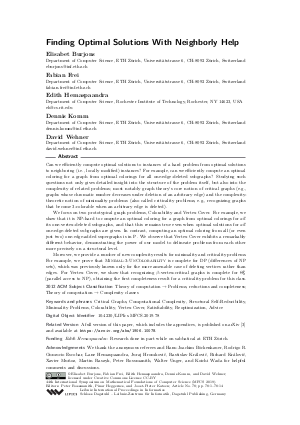Document# Finding Optimal Solutions With Neighborly Help

### Authors Elisabet Burjons, Fabian Frei, Edith Hemaspaandra, Dennis Komm, David Wehner## File

LIPIcs.MFCS.2019.78.pdf
• Filesize: 445 kB
• 14 pages

## Acknowledgements

We thank the anonymous referees and Hans-Joachim Böckenhauer, Rodrigo R. Gumucio Escobar, Lane Hemaspaandra, Juraj Hromkovič, Rastislav Královič, Richard Královič, Xavier Muñoz, Martin Raszyk, Peter Rossmanith, Walter Unger, and Koichi Wada for helpful comments and discussions.

## Cite As

Elisabet Burjons, Fabian Frei, Edith Hemaspaandra, Dennis Komm, and David Wehner. Finding Optimal Solutions With Neighborly Help. In 44th International Symposium on Mathematical Foundations of Computer Science (MFCS 2019). Leibniz International Proceedings in Informatics (LIPIcs), Volume 138, pp. 78:1-78:14, Schloss Dagstuhl - Leibniz-Zentrum für Informatik (2019)
https://doi.org/10.4230/LIPIcs.MFCS.2019.78

## Abstract

Can we efficiently compute optimal solutions to instances of a hard problem from optimal solutions to neighboring (i.e., locally modified) instances? For example, can we efficiently compute an optimal coloring for a graph from optimal colorings for all one-edge-deleted subgraphs? Studying such questions not only gives detailed insight into the structure of the problem itself, but also into the complexity of related problems; most notably graph theory’s core notion of critical graphs (e.g., graphs whose chromatic number decreases under deletion of an arbitrary edge) and the complexity-theoretic notion of minimality problems (also called criticality problems, e.g., recognizing graphs that become 3-colorable when an arbitrary edge is deleted). We focus on two prototypical graph problems, Colorability and Vertex Cover. For example, we show that it is NP-hard to compute an optimal coloring for a graph from optimal colorings for all its one-vertex-deleted subgraphs, and that this remains true even when optimal solutions for all one-edge-deleted subgraphs are given. In contrast, computing an optimal coloring from all (or even just two) one-edge-added supergraphs is in P. We observe that Vertex Cover exhibits a remarkably different behavior, demonstrating the power of our model to delineate problems from each other more precisely on a structural level. Moreover, we provide a number of new complexity results for minimality and criticality problems. For example, we prove that Minimal-3-UnColorability is complete for DP (differences of NP sets), which was previously known only for the more amenable case of deleting vertices rather than edges. For Vertex Cover, we show that recognizing beta-vertex-critical graphs is complete for Theta_2^p (parallel access to NP), obtaining the first completeness result for a criticality problem for this class.

## Subject Classification

##### ACM Subject Classification
• Theory of computation → Problems, reductions and completeness
• Theory of computation → Complexity classes
##### Keywords
• Critical Graphs
• Computational Complexity
• Structural Self-Reducibility
• Minimality Problems
• Colorability
• Vertex Cover
• Satisfiability
• Reoptimization

## Metrics

• Access Statistics
• Total Accesses (updated on a weekly basis)
0